# What's the minimum initial speed to score

Charlene

## Homework Statement

Alex Morgan is going to kick a soccer ball into the canadians goal during the 2015 world cup. Alex kicks the ball straight at the goal from 20.0 m away, and it launches off the ground at an 33.3 degree angle. Assume the goalie is busy faking an injury and ignore air resistance
a) what's the minimum initial speed that the soccer ball must have to reach the goal without touching the ground?
b)Assume Alex kicks the ball at the speed found in part a). if the canadian goalie takes 5.00 sec to get back into position to be able to stop the ball, does Alex score the goal?
c) the top of the net is 2.44 m off the ground, what is the maximum initial speed that the soccer ball can have (when the ball leaves her foot at this angle) and not miss scoring?

## Homework Equations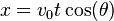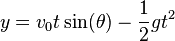## The Attempt at a Solution

a) so for the x displacement.
20=0+(vicosθ)t, so t= 20/(vicosθ)
for the y displacement do i not have to calculate it because it's going to be 0? i was not given the y value until part c) so now i don't know where to go about solving for the y displacement.

Are these the correct formulas to be looking at to try and solve this problem?

Staff Emeritus
Homework Helper

## Homework Statement

Alex Morgan is going to kick a soccer ball into the canadians goal during the 2015 world cup. Alex kicks the ball straight at the goal from 20.0 m away, and it launches off the ground at an 33.3 degree angle. Assume the goalie is busy faking an injury and ignore air resistance
a) what's the minimum initial speed that the soccer ball must have to reach the goal without touching the ground?
b)Assume Alex kicks the ball at the speed found in part a). if the canadian goalie takes 5.00 sec to get back into position to be able to stop the ball, does Alex score the goal?
c) the top of the net is 2.44 m off the ground, what is the maximum initial speed that the soccer ball can have (when the ball leaves her foot at this angle) and not miss scoring?

## Homework Equations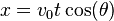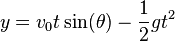## The Attempt at a Solution

a) so for the x displacement.
20=0+(vicosθ)t, so t= 20/(vicosθ)
for the y displacement do i not have to calculate it because it's going to be 0? i was not given the y value until part c) so now i don't know where to go about solving for the y displacement.
If the ball is kicked at an angle of 33.3 degrees to the horizontal, how can the y displacement be zero?
Are these the correct formulas to be looking at to try and solve this problem?
You have the correct formulas, you just need to apply them correctly to solve this problem.

As an aid to solution, draw a sketch of how the ball travels after being kicked until it reaches the goal.

Charlene
okay, so i found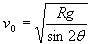but it says this can only be used when the initial and end elevation is the same, which in my problem this isn't going to be true because the end elevation is still in the air when it started on the ground.

so, relooking at the equations,
i have t= 20/(vicosθ)
and for the y,
0=(visinθ)t-1/2gt^2
(not too sure how to solve for t right this second)
then once they are both t= would i set the two equations = to each other and then solve for vi?

Staff Emeritus
Homework Helper
okay, so i foundbut it says this can only be used when the initial and end elevation is the same, which in my problem this isn't going to be true because the end elevation is still in the air when it started on the ground.

so, relooking at the equations,
i have t= 20/(vicosθ)
and for the y,
0=(visinθ)t-1/2gt^2
(not too sure how to solve for t right this second)
then once they are both t= would i set the two equations = to each other and then solve for vi?

In a), the problem asks for the minimum initial speed so that the kicked ball reaches the goal without touching the ground. Obviously, this initial speed is going to be greater than if the ball touches ground as it reaches the goal. The range formula can tell you what the absolute minimum initial speed is so that the ball hits ground as it reaches the goal.

In your equations above, there are several quantities which are fixed for this problem, like g and the angle θ.

Charlene
I asked one of my classmates for help on this problem and this is the solution he came up with. Isnt this incorrect tho because the range formula isnt keeping in mind that its not hitting the ground? Im honestly so lost and have an exam in a couple hours, hope to figure this out soon!

okay, the picture won't upload but he did the R= (vi^2sin2(theta))/g and solved to get vi=√20g/sin(66.6)=14.6

time to get there then is

b) 20m/(14.6)cos(33.3) = 1.64

c) vi= √ (g xf^2)/ (2cos^2(theta)(tan(theta)xf-yf)

Last edited:
Staff Emeritus